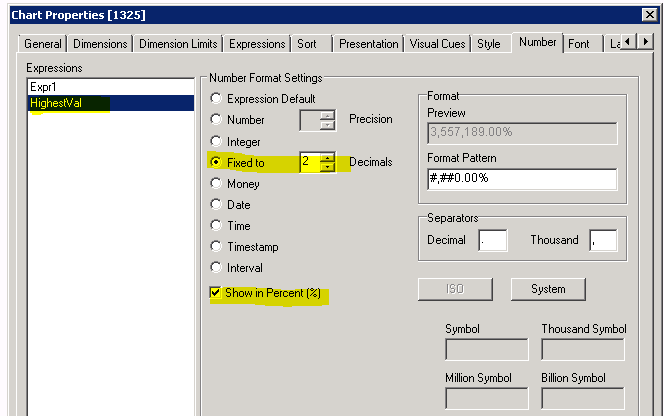# QlikView App Dev

Discussion Board for collaboration related to QlikView App Development.

Announcements
Support Cases coming to Qlik Community Oct. 4! Start chats, open cases, explore resources: READ DETAILS
cancel
Showing results for
Did you mean:Creator III

## Expression using the Max Value of another Column

Like Excel can take the largest value of a column range and use it to calculate a metric, I am looking to do this in Qlikview. I have been messing around with some ColumnNum & Max functions, but I can't seem to get it. The current expression1 is a sum(field1) which gives me their total dollars lets say... I am trying to create an expression to take that and divided by the person with the largest sum. This will give me their "percent to best". Maybe an expression that looks at that column/cell to the left and divides by the biggest number in that column?

I have provided a sample screenshot of what I am looking for. Any ideas?1 Solution

Accepted SolutionsChampion III

Try like using straight table

Dim: Dimension

Expressions:

= Sum(Expression1)

= Num(Sum(Expr)/max(TOTAL  Expr), '#,##0.00')*100 & '%'

you can set the %ge in Number tab of the chart properties like below2 RepliesChampion III

Try like using straight table

Dim: Dimension

Expressions:

= Sum(Expression1)

= Num(Sum(Expr)/max(TOTAL  Expr), '#,##0.00')*100 & '%'

you can set the %ge in Number tab of the chart properties like belowCreator III
Author

I thought I had almost that exact thing and it wasn't working... hmmm. Well, I was actually able to get it work with even a more simplistic version while formatting in the format tab itself.

= Sum(Field)/max(TOTAL  Field)Community Browser